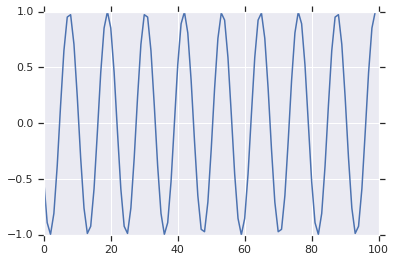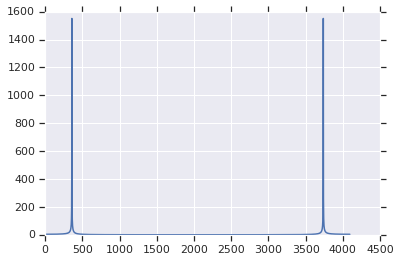# Digital Signal Processing

## DFT/FFT

DFT is a matrix multiplication, the naive implementation is $O(N^2)$. FFT is a much faster multiplication in $O(N \log N)$, which makes it practical.

## Digital Sinusoid Generators

Second-order digital resonator in Python:

fs = 8000  # sampling frequency
fo = 700   # output frequency
A = 1.0    # amplitude
w = 2*pi*fo/fs    # angular frequency of fo
c = 2.0 * cos(w)  # coefficient, controls the frequency, 1.70528
x = -A * sin(w)   # initial value, controls the amplitude and phase, -0.522498
y = 0.0
for ever:
emit(x)
x, y = c*x - y, x


First 100 data points:N = 4096
f = fft(signal[:N])
p = np.argmax(abs(f)[:N//2])
print(p*fs/N) => 699.21895 Hz


FFT: